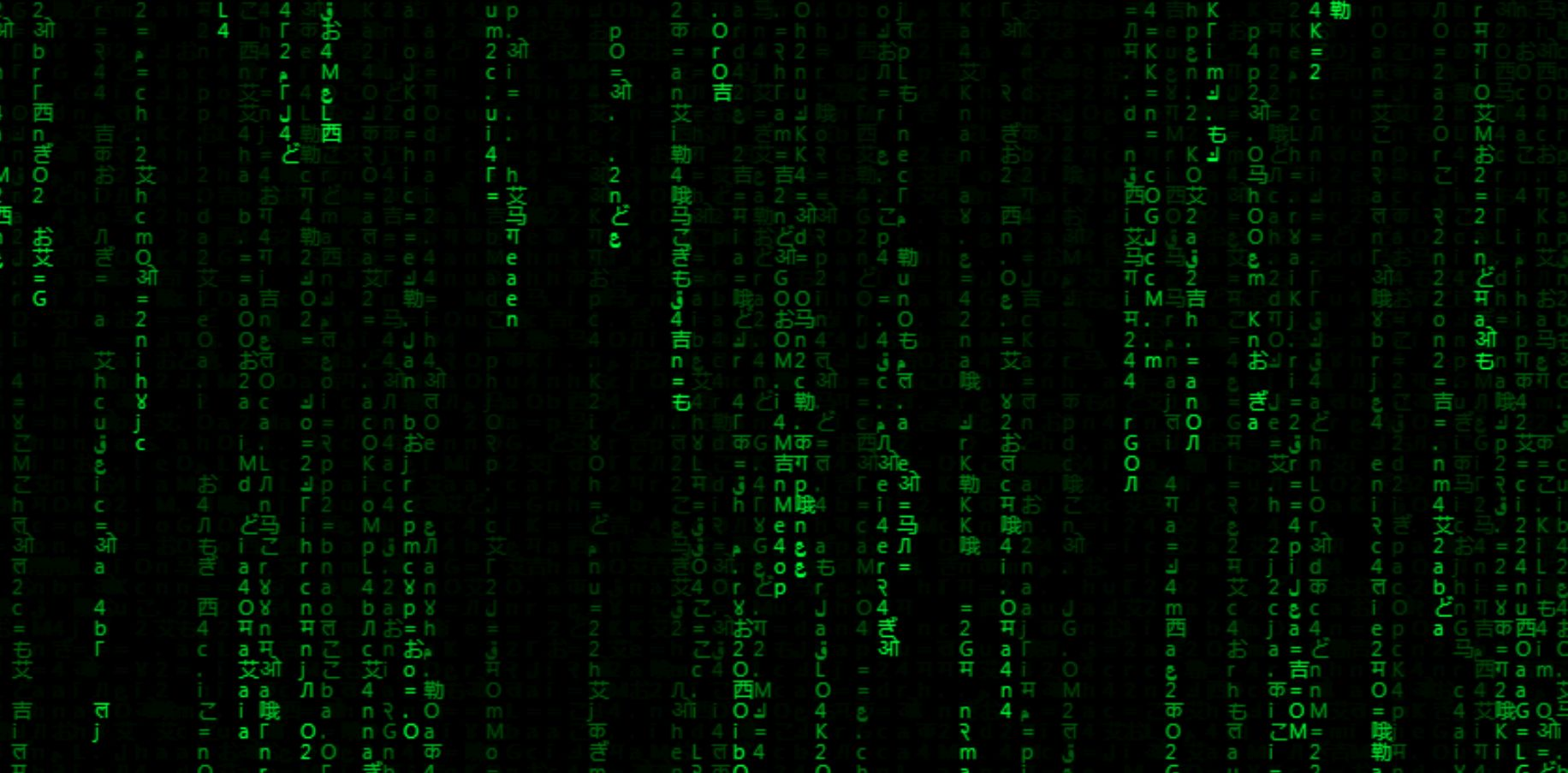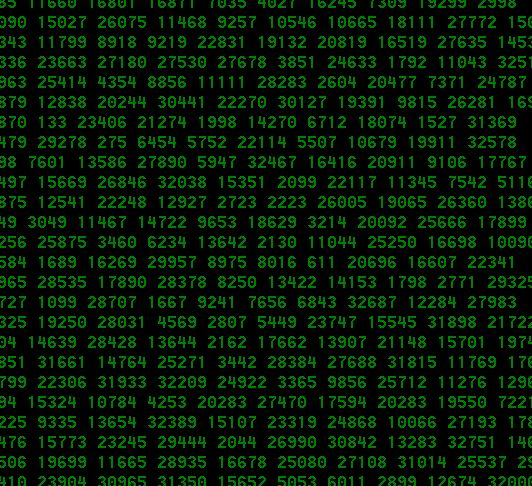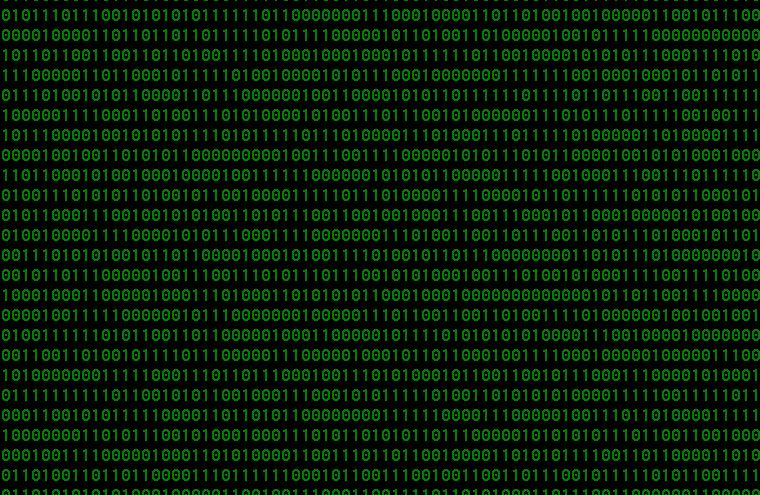﻿ How to create visual effect from the movie The Matrix, falling hieroglyphs

# How to create visual effect from the movie The Matrix, falling hieroglyphs

How to make the visual effect from the movie "The Matrix".

START MATRIXWelcome to the Matrix "falling" lines with hieroglyphs on screen.

For the web page don't forget
<html>
</html>

The markup looks like this:

<body style = "margin: 0; background: #000; overflow: hidden;">
<canvas> </canvas>
</body>

Let's initialize the canvas, context, canvas width and height.

We make the latter equal to the width and height of the browser window:

For the web page don't forget
<script>
</script>

const C = document.querySelector ("canvas"),
\$ = C.getContext ("2d"),
W = C.width = innerWidth,
H = C.height = innerHeight;

Create a string with characters that will be used for rendering (you can use any hieroglyphs, any alphabet, numbers, letters, symbols or even Cyrillic).

Convert this string to an array. Spaces give empty spaces in columns, with them the effect is more interesting:

const str = "24GLO.com=en 24ГЛО.КОМ=ru 24吉艾勒哦.西艾马=japan 24ぎどお.こおも=china २४गलओ.कओम=hindi 24قلعكعم=arabic",
matrix = str.split("");

Determine the font size, the number of columns and create an empty array. We will use this array to determine the y coordinate:

let font = 11,
//number of columns = canvas width/font size
col = W/font,
arr = [];

We fill the empty array with units according to the number of columns:

for (let i = 0; i <col; i++) arr [i] = 1;

Everything is ready for drawing. Let's get started:

function draw () {
//define the background color
//this color allows you to achieve the effect of a gradual fading of characters
\$.fillStyle = "rgba (0,0,0,.05)";

//fill the canvas with the selected color
\$.fillRect (0, 0, W, H);

//change the color for the font
\$.fillStyle = "#0f0";

//set font parameters
\$.font = font + "px system-ui";

//draw symbols
for (let i = 0; i <arr.length; i ++) {

//choose a random set of characters
let txt = matrix [Math.floor (Math.random () * matrix.length)];

//draw symbols
//move right and down
//fillText (character set, x coordinate = i value multiplied by font size, y coordinate = arr value multiplied by font size)
\$.fillText (txt, i * font, arr [i] * font);

//if "y" is greater than the canvas height or Math.random () produces more than 0.975 (the smaller this value, the more voids there will be on the screen), then go up (zero "y")
//this allows for the difference in rendering of individual columns
if (arr [i] * font> H && Math.random ()> 0.975) arr [i] = 0;

//increase y value
arr [i] ++;
}
}

We run the drawing function every 123 milliseconds (an arbitrary number):

setInterval (draw, 123);

Finally, when the window is resized, we reload the page:

The full code of Matrix for .html file (you can copy and create html web page):

<!DOCTYPE html>
<html>
<body style="margin: 0; background: #000; overflow: hidden;">
<canvas></canvas>

<script>
const C = document.querySelector("canvas"),
\$ = C.getContext("2d"),
W = (C.width = window.innerWidth),
H = (C.height = window.innerHeight);

const str = "24GLO.com=en 24ГЛО.КОМ=ru 24吉艾勒哦.西艾马=japan 24ぎどお.こおも=china २४गलओ.कओम=hindi 24قلعكعم=arabic",
matrix = str.split("");

let font = 11,
col = W / font,
arr = [];

for (let i = 0; i < col; i++) arr[i] = 1;

function draw() {
\$.fillStyle = "rgba(0,0,0,.05)";
\$.fillRect(0, 0, W, H);
\$.fillStyle = "#0f0";
\$.font = font + "px system-ui";
for (let i = 0; i < arr.length; i++) {
let txt = matrix[Math.floor(Math.random() * matrix.length)];
\$.fillText(txt, i * font, arr[i] * font);
if (arr[i] * font > H && Math.random() > 0.975) arr[i] = 0;
arr[i]++;
}
}

setInterval(draw, 123);
</script>
</body>
</html>

## The result (visual effect from the movie The Matrix, falling hieroglyphs) can be seen here.## Matrix effect (falling numbers) with NotepadTo make very simple Matrix effect with Notepad, just create file txt (then rename to .bat or .cmd) with following text:

@echo off
color 02
:start
echo %random% %random% %random% %random% %random% %random% %random% %random% %random% %random%
goto start

After that, double-click on it and get the result as in the picture above.

P.S. If you want to experiment, you can play with the flowers in the window. To do this, enter other numbers instead of 02 in the line “color”. For instance:

03 - blue numbers on a black background;
04 - red;
05 - lilac color;
06 - yellow;
07 - white;
08 - gray;
09 - blue;
10 - black numbers on a blue background;
11 - white numbers on a blue background;
12 - yellow numbers on a blue background;
etc.

## Matrix effect (falling 0 and 1) with NotepadTo make another very simple Matrix effect (falling 0 and 1) with Notepad, just create file txt (then rename to .bat or .cmd) with following text:

setlocal enabledelayedexpansion
echo off
title Matrix
color 02
cls
:continue
set line=
for /l %%i in (0,1,78) do (
set /a temp=!random!%%2
set line=!line!!temp!
)
set /a generate=!random!%
if %generate%==0 (color 0A) else (color 02)
echo %line%
goto continue

24glo.comContacts